## Abstract

This paper presents an experimental and numerical investigation on the mechanisms of damage onset and evolution in unidirectional carbon fibre reinforced thermoplastic (CFRTP) composites subjected to off-axis compressive loadings. A test fixture was designed to prevent buckling, splitting and end collapsing of specimens during test. A series of compression tests were conducted with specimens of various off-axis angles. Different failure mechanisms and nonlinear stress-strain responses were observed and studied. The fracture angles of the tested specimens were evaluated and analysed according to Puck’s theory. The off-axis compression failure envelope based on LaRC05 and Hashin criteria was presented using finite element analysis and compared to experimental results. It is shown that the LaRC05 criterion can provide accurate predictions for matrix cracking failure mode of unidirectional thermoplastic composites controlled by compression-shear combined stresses.

Keywords: Thermoplastic composites, off-axis compression, fibre kinking, failure envelope, fracture angle

## 1. Introduction

The increasing application of carbon fibre-reinforced thermoplastic composites (CFRTPs) in aerospace engineering has drawn significant attention to the compressive failure of unidirectional laminates which is generally recognized as a combined result of various mechanisms. It is one of the most challenging tasks to comprehensively describe these behaviours. A considerable amount of research on off-axis compression tests has been conducted in the past decades. Failure modes like fibre splitting, interface decohesion, fibre kinking and matrix shear failure have been experimentally observed and further explained in several theoretical studies [1,2,3]. The fibre kinking is a compressive fracture mechanism of fibre reinforced composites (FRP) that caused by micro-buckling of fibres, and was firstly reported and modelled by Argon  and Budiansky . They affirmed that the initial misalignment is the cause of fibre kinking mode. Further, Budiansky and Fleck  took the strain hardening effect of matrix into consideration when analysing the fibre kinking. Lee and Waas  studied the compressive strength and failure modes of unidirectional glass fibre reinforced polymer (GFRP) and carbon fibre reinforced polymer (CFRP). A finite element (FE) model was presented to study the effect of initial misalignment angles of the fibres. Pimenta et al.  studied the initiation and propagation of kink-bands through compression tests of notched unidirectional CFRP plates. Ueda et al.  investigated the kink-band formation of CFRP using X-ray micro-CT. Failure model proposed by Pinho et al.  includes the fibre kinking theory that can accurately predict the compressive failure of fibre reinforced unidirectional composite. The splitting was initially observed by Piggott and Harris  through compression test of unidirectional GFRP composites. Later, splitting was identified in compressive failure mechanisms of carbon fibre reinforced composites by Oguni and Ravichanran . Lee and Waas  investigated the effect of different fibre volume fractions on the compressive failure mechanisms of unidirectional glass fibre and carbon fibre composites. A combined fibre kinking and splitting failure mode was reported at different fibre volume fractions. Prabhakar et al.  studied the relationship between fibre kinking and fibre splitting of unidirectional composites and concluded that the splitting mode dominates the compressive strength. Yerramalli et al.  investigated the fracture mechanics of glass fibre and carbon fibre composites under combined compressive and shear stresses, and suggested that both matrix splitting and fibre kinking contribute to the composite failure.

The compressive strength of unidirectional composite is also affected by transverse shear behaviour . The mingled shear stress during the compression of unidirectional CFRP composites was investigated by Vogler et al. . Their experiments showed that the shear field introduces extra fibre rotation and bending. Tsai and Sun  conducted off-axis compressive tests of CFRP specimens under different strain-rate. In-plane shear stress was extracted from the compounded stress field of the off-axis compression. Their results show that the in-plane shear strength plays a significant role in the specimen failure.

Several models based on physical mechanisms were proposed to reflect the different failure modes and correlate well with experimental phenomena [16,17]. Zhou et al.  investigated the failure mechanism of GFRP composites under off-axis compressive loading conditions. Fibre kinking, fibre buckling and matrix cracking were observed in the specimens with different off-axis angles. González and Llorca  investigated the transverse compression mechanisms of unidirectional GFRP and CFRP composites. Micromechanical simulation indicated that the interface decohesion and shear failure are the two main failure modes. Matsuo and Kageyama  developed a modified kink band model that considers transverse tension and shear stresses within the kink band area. Thomson et al.  conducted 0°, 3°, 6°, 10° and 15° off-axis compression experiments using unidirectional IM7/8852 specimens with different designs. Results suggest that dog-bone specimens will cause stress contribution at the changing area. It is also reported that accurate measurements were hard to achieve since the specimens are sensitive to boundary conditions. Lee and Soutis  evaluated the compressive strength of unidirectional CFRP composites using compression fixtures that clamped both ends of the specimens, results show the over clamping of specimens and stress concentration at the gripping area have severe effect on the compression strength. Misaligned fibres and wavy plies also affect the compressive failure stress. However, the mechanical behaviour of unidirectional composites under compression, which is vital for understanding the fibre kinking and splitting mechanics, has not been investigated thoroughly due to the difficulty of experiment conducting. Thus, to obtain reliable measurements of off-axis compression strength and investigate the failure mechanisms of unidirectional composites, carefully designed experiments need to be conducted.

The purpose of this paper is to study the compressive behaviour of carbon fibre reinforced thermoplastic composite through compression tests of off-axis specimens, which produce a set of combined stress states with uniaxial load and lead to different failure modes. The experimental results were evaluated and failure modes were analysed.

## 2. Materials and methods

Off-axis specimens were cut at different orientations from a 24-ply Cetex® TC1200 PEEK/AS4 carbon/thermoplastic unidirectional laminate. The volume fraction of the resin is 34% and the nominal thickness of each layer is 0.14mm. Up to five off-axis angles $\theta =$ 15°, 30°, 45°, 60°, 75°, along with two special angles $\theta$° (longitudinal compression) and 90° (transverse compression) were selected to bring forth different failure modes and investigate their transition with each other. The sketch of the off-axis compression test specimen is shown in Figure 1. Nominal dimensions of the specimens are 36mm(H)$\times$12mm(W)$\times$3mm (T). The area of the evaluation section is 12mm$\times$12mm. Parallelism tolerances of all opposing surfaces was strictly inspected, and the loading end surfaces were well polished before tests.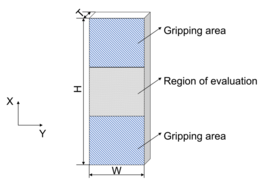Figure 1. Schematic of off-axis specimens

To ensure stable loading to the small size specimens and eliminate potential off-axis bending along the longitudinal direction, a special fixture was designed as shown in Figure 2. When conducting the off-axis compression test, the specimen was placed in the centre of the fixture with the top and bottom ends being supported by two loading blocks. The gripping areas of the specimen were clamped by two pairs of wedged clamping blocks to prevent the specimen from buckling and provide compressive loading through friction between the surfaces. However, excessive gripping forces could cause specimen gripping area collapse and specimen over-constrained in the transverse direction. Hence, set screws are used to control the clamping forces to provide enough lateral support to the specimen while not introduce additional restrains.

 Figure 2. (a) Off-axis compression test fixture. (b) Sectional view of off-axis compression test fixture. (c) Off-axis compression experiment setup

The compression tests were carried out using a DDL100 electronic universal testing machine with a 100kN load cell. The fixture was settled between upper and lower compression platens which are self-adjusted parallel with each other via a pair of contact spherical surfaces in the upper platen set. Each specimen was loaded at a speed of 0.5mm/min. In-plane strain fields of the specimen were captured and determined by means of the digital image correlation (DIC) method via a non-contact optical system ARAMIS 4M. The strain gauges were also attached onto the back surface of the specimens to monitor the axial strain.

In preparation for DIC measurement, the specimens were first painted white and then a random black spray pattern was applied on the surface. A 35mm lens with distance ring was used for the camera of the DIC system and was positioned at around 0.3m away from specimen surface to capture speckle pattern images at 2352$\times$1728 pixels2 resolution. The recording frequency was set at 1 frame per second. The axial stress was obtained from the applied load divided by the specimen cross-sectional area.

In order to avoid error coming from the vicinity of specimen edges, a rectangular central area of the size of $10\times 10$ mm2 was selected as the region of interest, in which the displacement field and strain data was measured to represent overall deformations of the specimen. According to Reu , the subset size was selected as $21\times 21$ pixels2 to cover enough pattern features. The step size of $9\times 9$ pixels2 was chosen to ensure sufficient spatial resolution. The virtual strain gauge (VSG) approach was used to calculate the strain.

## 3. Results and discussion

### 3.1. Faliure modes

Figure 3 illustrates the failure modes of different specimens under compressive loading. For each specimen, the photographs of the front and back surfaces are taken to evaluate the failure mechanisms. No splitting and end collapse occurred in the 0° and 15° specimens, which indicates that the current test fixture can prevent the specimens from fibre splitting and end collapsing. Hence, accurate compressive strengths of off-axis specimens can be obtained through this test method. In the case of 30° off-axis angle, the fracture surface appeared to be scarcely slanted to the through thickness direction, indicating the existence of in-plane shear failure mode, also referred to as splitting in some literatures. A closer inspection of the fractured specimens revealed that kink-bands have developed from the loading ends of 0° and 15° specimens. On the contrary, no sign of fibre kinking was found in the other specimens. This observation suggests that the transition from kinking to splitting occurred between 15° to 30°.

For the specimens with 45°, 60° and 75° off-axis angles, compression-shear mixed mode matrix failure was witnessed with the fracture surfaces developed along the fibre direction. For transverse compression (90°), specimens normally separated into several pieces.

### 3.2. Mechanical responses

To evaluate the accuracy of the data collected by DIC, the stress-strain curves of specimens with 60° off-axis angles obtained from the strain gauges and DIC measurements were plotted together in Figure 4. Comparison of the two methods shows that results from strain gauges are slightly smaller. This is reasonable since the measuring grids of a strain gauge is mounted on a carrier foil and then attached to the specimen surface using glue, this insertion between measuring grids and specimen surface brings error to the evaluated strain fields.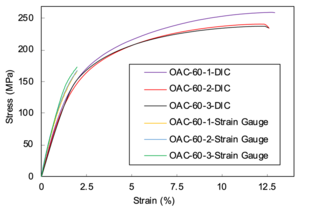Figure 4. Comparison of strain data collected from DIC and strain gauge for 30° off-axis compression test

Typical stress-strain responses for different off-axis specimens are plotted in Figure 5. It can be seen that except for 0° and 15° off-axis specimens, all the other specimens exhibit apparent non-linear deformation before failure. The mechanical response of 0° unidirectional compression is linear until final failure, all three specimens show good correlation. However, the 15° off-axis compression stress-strain relationships show obvious variation, a slight nonlinearity was observed in the curves. The maximum average strains of 0° and 15° specimens are similar, while the average strength of axial compression specimens is 350 MPa higher than 15° off-axis compression specimens.

For the 30° to 90° off-axis compression specimens, the initial modules were linear until strain hardening stages emerged. Final failures were reached after significant plastic deformation stage. The 45° off-axis specimen has a highest failure strain of 14% while the 75° off-axis compression strain is around 9%. Compared to thermoset composites , the thermoplastic composite exhibits prominent plastic deformation and much higher failure strain.

 Figure 5. Stress-strain curves of off-axis specimens under compression. (a) 0° axial compression. (b) 15° off-axis compression. (c) 30° off-axis compression. (d) 45° off-axis compression. (e) 60° off-axis compression. (f) 75° off-axis compression. (g) 90° transverse compression

### 3.2. Visualization of displacements and strains

Full field displacements and strains of the region of interest were analysed using DIC method. To eliminate errors from free edges and clamping, areas near the gripping lines and edges were not included.

Figure 6 illustrates the displacement and strain fields of a 0° on-axis compression specimen before fibre kinking failure. The transverse displacements contours distribution is not parallel to the fibre direction, show a nonuniformly deformation across the width of the specimen. The longitudinal displacements are evenly placed over the specimen width. The strain fields in both x and y directions are consistent in the centre area of the specimen. Strain concentration of ${\textstyle {\epsilon }_{x}}$ on the upper right of the inspection region has arisen, while no damage was discovered at this position when inspecting the specimen after the test. The strain concentration area spotted in ${\textstyle {\epsilon }_{y}}$ strain fields match the region where fibre kinking happened.

 Figure 6. Displacement and strain fields contour plots of 0° axial compression specimen. (a) Displacement in $x$ direction. (b) Displacement in $y$ direction. (c) Strain in $x$ direction. (d) Strain in $y$ direction

Figure 7 shows the axial and transverse displacement and strain fields of a 15° off-axis specimen prior to failure initiation. The displacement fields contour plot shows clear patterns before failure. The transverse displacement gradient changes perpendicular to the fibre direction, while the axial displacement gradient varies parallel to the fibres. The strain fields are not evenly distributed over the specimen. The transverse strain fields demonstrate high level of strain concentration in the area where fibre kinking occurred. The contour plot of axial strain fields indicates high strain gradient variation along the width of the specimen.

 Figure 7. Displacement and strain fields contour plots of 15° off-axis specimen. (a) Displacement in $x$ direction. (b) Displacement in $y$ direction. (c) Strain in $x$ direction. (d) Strain in $y$ direction

The illustrated displacement fields in Figures 8(a) and (b) indicates that the displacement gradients were inerratic. However, the strain was not evenly distributed. Extreme strain concentrations are presented along the cracking route. The highest level of strain concentration indicates the matrix damage initiation site.

 Figure 8. Displacement and strain fields contour plots of 30° off-axis specimen. (a) Displacement in $x$ direction. (b) Displacement in $y$ direction. (c) Strain in $x$ direction. (d) Strain in $y$ direction

Figure 9 depicts the displacement and strain fields before matrix cracking failure of a 45° off-axis specimen. The displacement fields are conformably distributed along the fibre direction. The strain fields are inconsistent with strain concentration points are scattered on the specimen surface. Highest strain values are distributed along the matrix cracking routine for both transverse and axial strain fields. High positive and negative strain values are commingled along the fracture zone in both ${\textstyle {\epsilon }_{xx}}$ and ${\textstyle {\epsilon }_{yy}}$ fields. This could be induced by local matrix micro-cracking damage.

 Figure 9. Displacement and strain fields contour plots of 45° off-axis specimen. (a) Displacement in $x$ direction. (b) Displacement in $y$ direction. (c) Strain in $x$ direction. (d) Strain in $y$ direction

The displacement and strain fields of a 60° off-axis specimen is shown in Figure 10. High strain gradients along the fibre direction were captured prior to failure. Although some strain concentration areas are coincident with matrix cracking failure position, specimen surface with no fracture also exhibits strain concentration.

 Figure 10. Displacement and strain fields contour plots of 60° off-axis specimen. (a) Displacement in $x$ direction. (b) Displacement in $y$ direction. (c) Strain in $x$ direction. (d) Strain in $y$ direction

As the off-axis angle inclined to 75°, the prior to failure transverse displacement field shown in Figure 11 variates prominently. Large deformation gradient appears in coincidence with failure location. The ${\textstyle {\epsilon }_{y}}$ strain fields contain significant concentrations along the matrix fracture path. The severity of the strain concentration denotes the matrix damage accumulation level. The highest strain concentration level represents the failure initiation location. The ${\textstyle {\epsilon }_{x}}$ strain patterns show local variation with strain concentrations scattered over the specimen surface. The peak values are in coincide with the failure point.

 Figure 11. Displacement and strain fields contour plots of 75° off-axis specimen. (a) Displacement in $x$ direction. (b) Displacement in $y$ direction. (c) Strain in $x$ direction. (d) Strain in $y$ direction

The displacement and strain fields of a 90° on-axis transverse compression specimen is shown in Figure 12. The specimen exhibits consistent displacement arrangement. Apparent strain concentrations of ${\textstyle {\epsilon }_{y}}$ appeared in accordance with final fracture site. Cracks would be originated from these peaks of strain values to form the fracture surface.

 Figure 12. Displacement and strain fields contour plots of 90° off-axis specimen. (a) Displacement in $x$ direction. (b) Displacement in $y$ direction. (c) Strain in $x$ direction. (d) Strain in $y$ direction

### 3.3. Fractography

Although vast amount of fractography investigations were carried out for thermoset composites , few research was emphasised on thermoplastic composites [26,27]. Some researchers have conducted fractography studies of thermoplastic composites under mode I and II fractures. The plastic behaviour of the thermoplastic matrix and its interaction with fibres during compressive/shear combined loadings still need fractographical analysis to reveal its failure mechanism.

SEM observations were conducted on all tested specimens at the in-plane direction, which is perpendicular to the thickness direction. Due to the different scales of the fracture surface, SEM images were taken in vary magnifications and were shown in Figure 13.

 Figure 13. SEM images of the fracture surfaces of on-axis and off-axis specimens under compressive loads. (a) 0° axial compression. (b) 15° off-axis compression. (c) 30° off-axis compression. (d) 45° off-axis compression. (e) 60° off-axis compression. (f) 75° off-axis compression. (g) 90° on-axis transverse compression

Clear fibre kink-band can be identified for 0° and 15° off-axis specimens, indicating thoroughly developed fibre kinking failure mechanism. From the 15° off-axis specimen graphic, severe fibre buckling without fibre fracture at the boundary of the kink-band can be seen. Fibre kinking in both in-plane and out-of-plane directions were observed, indicates a three-dimensional failure mode. The kink-bands of 0° and 15° specimens share the same pattern. Generally, with sufficient lateral support from the matrix, fibres will fracture under shear stress instead of micro-buckling . The ductile behaviour of the thermoplastic matrix often exhibits large plastic deformation and reduces lateral support to the fibres, micro-buckling was then triggered. Therefore, the scale of the kink band can be related to the mechanical parameters of the matrix. Compared to thermoset composites, the longer kink band length implies a relatively ductile matrix. The parameters that control the fibre kinking formation can be measured, which are the kink-band width ${\textstyle d}$ and kink-band incline angle ${\textstyle \alpha }$ as shown in Figure 14. The measured average kink-band width and incline angle are 911.96 μm and 27.28°, respectively.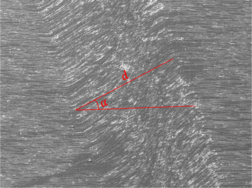Figure 14. Measure of the kind-band width and incline angle

For 30° off-axis compression, failure was dominated by the matrix fracture. A parabola fracture pattern that existed in the PEEK matrix region, indicating a fracture growth direction of from the apex towards the enclosed area of the parabola. Fibre was fractured and debris was generated due to the compressive loading along the fibre direction. Matrix plastic deformation caused by the shear force indicates a ductile damage mechanism of thermoplastic matrix. The ductility of the matrix also causes significant drawing around the interface of the fibres. The matrix draws on the interface and peels away the resin, resulted in debonding and nodules over the fibres . Voids were nucleated, grew and coalesced under high plastic deformation and resulted in fibrillation . With the combined compressive stress, the shear fractured surface was abraded and shown smeared morphology. Scarps were emerged by the coalescence of multiple matrix fractures initiated by shearing.

The SEM inspection of 45° specimens show prominent matrix plastic deformation, indicating a plastic shear damage mode of the matrix. Also, fibrillation induced by noticeable plasticity can be spotted. The higher magnification SEM images of the 45° off-axis compression fracture surface show interface debonding with nodules on the surface that caused by the ductile drawing of the thermoplastic resin. Surface debris was produced as a results of matrix shear/compression mixed failure.

On the fracture surface of 60° off-axis specimens, fibre splitting and fractured short fibres were generated by the compression/shear combined stresses. A closer inspection at the fractured surface shows fibrillations and smeared matrix, suggest void deformation and coalescence mechanism under high-level of plastic deformation. Ductile drawing on the surface of fibres was left due to slow crack growth rate. Debris on the fracture surface were generated during the fracture of the matrix.

The failure modes of 75° and 90° off-axis compression tests have similar features. A lot of fibre fractures and splitting were produced on the fracture surface. The matrix exhibits highly plastic deformation and were drawn from the fibre surface. Debris and nodules were left on the surface of fibres and matrix after compression dominated fracture.

Compared to the shear/compression fracture of brittle thermoset epoxies, which the dominant fracture feature is cusps formation mechanism with companion cleavages, riverlines and textured microflows, no shear cusp was detected for thermoplastic composites. The toughened thermoplastic material has higher fracture energy and more ductility, leads to plastic deformation and void coalescence dominated failure mechanisms. Riverlines that often seen in brittle fractured polymers were also not found. Therefore, it is hard to deduce the fracture direction of thermoplastic matrix.

### 3.4. Failure envelope

According to Puck and Schurmann , when the T300/914C carbon fibre reinforced epoxy unidirectional laminate is under transverse compression (${\textstyle {\sigma }_{22}<0}$) and in-plane shear, the matrix cracks will initiates and propagates along the surfaces that parallel to the fibre direction as shown in Figure 15(a). The angle α between the fracture surface and the through thickness direction varies under different stress states as shown in Figure 15(b). For most thermoset composites, as the value of ${\textstyle \left|{\sigma }_{22}\right|}$ rises, the fracture surface angle α increases from 0° to 53°.

 Figure 15. (a) Angle between the fracture surface and the through thickness direction. (b) the change of fracture angle α under different stress conditions 

The failure modes of the CFRTP specimens that tested also shows similar features of the fracture surface, as shown in Figure 16. Figure 17 shows the curve of the fracture angle vs. off-axis angle. The angle α increased to 12.3° for the 45° off-axis specimens before zooming to 54.75°, and the maximum fracture surface angle was attained as 59.16° for the 90° compression case.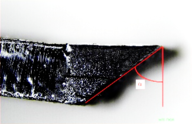Figure 16. Fracture angles of the off-axis compression specimensFigure 17. Fracture angles vs. off-axis angles

According to the Hashin failure criterion , for plane stress condition, and combining the stress transformation equations, the values of ${\textstyle {\sigma }_{22}}$ and ${\textstyle {\tau }_{12}}$ at compressive failure for off-axis unidirectional laminates are:

 ${\sigma }_{22}=X{\sin }^{2}\theta$
(1)
 ${\tau }_{12}=X\sin \theta \cos \theta$
(2)

where $X$ is the strength upon failure:

 $X={\frac {{S}_{L}{Y}_{C}}{\sin \theta {\sqrt {{S}_{L}^{2}{\sin }^{2}\theta +{Y}_{C}^{2}{\cos }^{2}\theta }}}}$
(3)

where ${\textstyle {S}_{L}}$ is the in-plane shear strength obtained from experiments, ${\textstyle {Y}_{C}}$ is the transverse compressive strength, ${\textstyle \theta }$ is the off-axis angle of the fibers, ${\textstyle {\sigma }_{22}}$ and ${\textstyle {\tau }_{12}}$ are the transverse normal stress and in-plane shear stress upon failure, respectively.

The LaRC05 theory is considered as one of the most accurate failure criteria according to the second World-Wide Failure Exercise (WWFE-II). This failure criterion is proposed as a physically based model that characterises the failure modes of composites in three types: matrix failure, fibre compression failure and fibre tension failure.

For matrix failure mode (MF):

 ${\left({\frac {{\tau }_{T}}{{S}_{T}^{is}-{\eta }_{T}{\sigma }_{n}}}\right)}^{2}+{\left({\frac {{\tau }_{L}}{{S}_{L}^{is}-{\eta }_{L}{\sigma }_{n}}}\right)}^{2}+$${\left({\frac {\left\langle {\sigma }_{n}\right\rangle }{{Y}_{T}^{is}}}\right)}^{2}=$$1$
(4)

where ${\textstyle \left\langle {\sigma }_{n}\right\rangle =({\sigma }_{n}+}$$\left|{\sigma }_{n}\right|)/2$, ${\textstyle {S}_{T}^{is}}$, ${\textstyle {S}_{L}^{is}}$ and ${\textstyle {Y}_{T}^{is}}$ are the in-situ transverse shear strength, in-situ longitudinal shear strength and in-plane transverse tension strength, respectively. The fracture surface stress components ${\textstyle {\sigma }_{n}}$, ${\textstyle {\tau }_{T}}$ and ${\textstyle {\tau }_{L}}$ are given by Puck :

 $\left\{{\begin{matrix}{\sigma }_{n}={\frac {{\sigma }_{2}+{\sigma }_{3}}{2}}+{\frac {{\sigma }_{2}-{\sigma }_{3}}{2}}\cos 2\alpha +{\tau }_{23}\sin 2\alpha \\{\tau }_{T}=-{\frac {{\sigma }_{2}-{\sigma }_{3}}{2}}\sin 2\alpha +{\tau }_{23}\cos 2\alpha \\{\tau }_{L}={\tau }_{12}\cos \alpha +{\tau }_{31}\sin \alpha \end{matrix}}\right.$
(5)

where ${\textstyle \alpha }$ is the fracture surface angle that can be determined numerically or experimentally, ${\textstyle {\alpha }_{0}}$ represents the fracture surface angle of transverse compression of unidirectional plates.

For fibre compression kinking failure mode:

 ${\left({\frac {{\tau }_{23}^{m}}{{S}_{T}^{is}-{\eta }_{T}{\sigma }_{2}^{m}}}\right)}^{2}+$${\left({\frac {{\tau }_{12}^{m}}{{S}_{L}^{is}-{\eta }_{L}{\sigma }_{2}^{m}}}\right)}^{2}+$${\left({\frac {{\left\langle {\sigma }_{2}^{m}\right\rangle }_{+}}{{Y}_{T}^{is}}}\right)}^{2}=1,\,{\sigma }_{1}\leq -{\frac {{X}_{c}}{2}}$
(6)

where ${\textstyle {\eta }_{T}\,}$ and ${\textstyle {\eta }_{L}}$ are the transverse shear and longitudinal shear friction coefficient, and are defined as:

 ${\eta }_{T}=-{\frac {1}{\tan 2{\alpha }_{0}}}$, ${\textstyle {\eta }_{L}={S}_{L}{\frac {{\eta }_{T}}{{S}_{T}}}}$
(7)

where ${\textstyle {S}_{L}}$ and ${\textstyle {S}_{T}}$ are the longitudinal and transverse shear strength of unidirectional composites on the fracture surface:

 ${S}_{T}={\frac {{Y}_{C}}{2\tan {\alpha }_{0}}}$
(8)

where ${\textstyle {Y}_{C}}$ is the transverse compression strength and ${\textstyle {\alpha }_{0}}$ is the fracture plane angle to the thickness direction under transverse compression.

For fibre compression splitting failure mode:

 ${\left({\frac {{\tau }_{23}^{m}}{{S}_{T}^{is}-{\eta }_{T}{\sigma }_{2}^{m}}}\right)}^{2}+{\left({\frac {{\tau }_{12}^{m}}{{S}_{L}^{is}-{\eta }_{L}{\sigma }_{2}^{m}}}\right)}^{2}+$${\left({\frac {{\left\langle {\sigma }_{2}^{m}\right\rangle }_{+}}{{Y}_{T}^{is}}}\right)}^{2}=1,\,-$${\frac {{X}_{c}}{2}}\leq {\sigma }_{1}\leq 0$
(9)

For fibre tension failure, the maximum stress criterion was adopted:

 ${\frac {{\left\langle {\sigma }_{1}\right\rangle }_{+}}{{X}_{T}}}=1$
(10)

where ${\textstyle {X}_{T}}$ is the longitudinal tension strength.

The in-situ strengths for unidirectional composites are calculated as:

 $\left\{{\begin{matrix}{Y}_{T}^{is}={Y}^{T}\\{S}_{Y}^{is}={S}^{Y}\\{S}_{T}^{is}={Y}_{C}\cos {\alpha }_{0}\sin {\alpha }_{0}+\displaystyle {\frac {\cos {\alpha }_{0}}{\tan 2{\alpha }_{0}}}\end{matrix}}\right.$
(11)

where ${\textstyle {Y}_{C}}$ is the transverse compression strength, ${\textstyle {Y}^{T}}$ is the transverse tension strength and ${\textstyle {S}^{Y}}$ is the shear strength. The value of ${\textstyle {\eta }_{L}}$ can be deduced from off-axis compression test. All material properties that required for the numerical simulation using LaRC05 criterion is listed in Table 1.

Table 1. Material parameters of AS4/PEEK
${\textstyle {\mathit {\boldsymbol {E}}}_{\mathit {\boldsymbol {11}}}}$ (GPa) ${\textstyle {\mathit {\boldsymbol {E}}}_{\mathit {\boldsymbol {22}}}}$ (GPa) ${\textstyle {\mathit {\boldsymbol {E}}}_{\mathit {\boldsymbol {33}}}}$ (GPa) ${\textstyle {\mathit {\boldsymbol {G}}}_{\mathit {\boldsymbol {12}}}}$ (GPa) ${\textstyle {\mathit {\boldsymbol {G}}}_{\mathit {\boldsymbol {23}}}}$ (GPa) ${\textstyle {\mathit {\boldsymbol {G}}}_{\mathit {\boldsymbol {13}}}}$ (GPa) ${\boldsymbol {\upsilon }}_{\mathit {\boldsymbol {12}}}$ ${\boldsymbol {\upsilon }}_{\mathit {\boldsymbol {13}}}$ ${\boldsymbol {\upsilon }}_{\mathit {\boldsymbol {23}}}$ ${\textstyle {\mathit {\boldsymbol {X}}}_{\mathit {\boldsymbol {T}}}}$ (MPa)
133 9.08 9.08 4.31 3.44 4.31 0.3 0.3 0.5 2001
${\textstyle {\mathit {\boldsymbol {X}}}_{\mathit {\boldsymbol {C}}}}$ (MPa) ${\textstyle {\mathit {\boldsymbol {Y}}}_{\mathit {\boldsymbol {T}}}}$ (MPa) ${\textstyle {\mathit {\boldsymbol {Y}}}_{\mathit {\boldsymbol {C}}}}$ (MPa) ${\textstyle {\mathit {\boldsymbol {S}}}_{\mathit {\boldsymbol {L}}}}$ (MPa) ${\textstyle {\mathit {\boldsymbol {S}}}_{\mathit {\boldsymbol {T}}}}$ (MPa) ${\mathit {\boldsymbol {\varphi }}}_{\mathit {\boldsymbol {0}}}$ ${\mathit {\boldsymbol {\alpha }}}_{\mathit {\boldsymbol {0}}}$ ${\mathit {\boldsymbol {\eta }}}_{\mathit {\boldsymbol {L}}}$ ${\mathit {\boldsymbol {\eta }}}_{\mathit {\boldsymbol {T}}}$
816 104 201 72.4 65.6 4.5° 59° 0.59 0.54

To predict the failure locus of the tested off-axis thermoplastic composites, a 3-dimensional finite element model was developed to assess the behaviours under different stress states and failure criteria. The model was built in Abaqus with dimensions the same as the specimen (Figure 1). 8-node quadratic element with reduced integration (C3D8R) was used to discretize the model. A series of numerical simulations of off-axis compression failure using Hashin and LaRC05 criteria were carried out.

The ${\textstyle {\sigma }_{22}-{\tau }_{12}}$ failure envelope in the second quadrant based on numerical predictions are plotted in Figure 18, and are compared to the results obtained from off-axis tests. It can be seen from the experimental results that the transverse compression leads to increased shear strength, which agrees with the Puck’s theory. The predictions based on Hashin failure criterion are over conservative refer to the experimental results. As a contrast, the LaRC05 failure criterion  that takes the in-situ effects into consideration and included fibre-kinking mechanism in the fibre failure modes, have shown more accurate prediction than Hashin’s criteria for the compression-shearing coupling scenarios. However, underestimation still exist for the strength predictions of LaRC05 criterion when the off-axis angle was under 15°. The reasons would be that the LaRC05 criterion not accurately predicted the fibre-kinking failure mode when the matrix toughness and plastic deformation increases.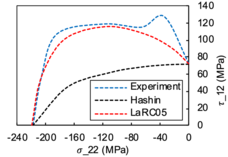Figure 18. Off-axis compression failure envelope

## 4. Conclusions

An experimental investigation of unidirectional carbon fibre reinforced thermoplastic composites AS4/PEEK under off-axis compression was conducted using DIC technique. Conclusions can be drawn as the following:

(1) With a new designed off-axis compression test fixture, fibre splitting and end collapsing can be avoided during the off-axis compression tests;

(2) The material matrix exhibits evident nonlinearity under mixed shear-compression stress state, so that a constitutive model should be proposed in the further study;

(3) Fibre kinking were observed in the specimens with small off-axis angles. The trend of the fracture modes and the fracture angles with through the thickness direction vs. off-axis angles were also observed and discussed. The matrix shear dominates the failure as the angle increases and high level of plastic deformation of the thermoplastic matrix was observed;

(4) The compression failure envelope for different shear-compression combinations predicted based on Hashin criterion is too conservative compared to experimental results, while the LaRC05 criterion made excellent predictions when the off-axis angle is larger than 15°.

Author contributions: Conceptualization: Y. Ma, Y. Li and L.Liu; methodology: Y. Ma; software: Y. Ma; validation: Y. Li; formal analysis: L. Liu; investigation: Y. Ma, L. Liu; resources: Y. Li; data curation: Y. Ma; writing—original draft preparation: Y. Ma; writing—review and editing: Y. Li; visualization: L. Liu; supervision: Y. Li; project administration: Y. Li. All authors have read and agreed to the published version of the manuscript.

### Document informationPublished on 07/02/23
Accepted on 02/02/23
Submitted on 29/06/22

Volume 39, Issue 1, 2023
DOI: 10.23967/j.rimni.2023.02.001

### Document Score0

Views 12
Recommendations 0